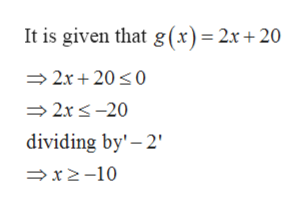# Use the function f(x)=x^2-4 and g(x)=2x+20 and answer (a)(a) Solve g(x)<=0.What is the correct answer in interval notation?

Question
34 views

Use the function f(x)=x^2-4 and g(x)=2x+20 and answer (a)

(a) Solve g(x)<=0.

What is the correct answer in interval notation?

check_circle

Step 1

Given: -

Step 2

To find: -

Step 3

Calculation...help_outlineImage TranscriptioncloseIt is given that g(x)= 2x + 20 2x 200 2xs-20 dividing by' 2 x2-10 fullscreen

### Want to see the full answer?

See Solution

#### Want to see this answer and more?

Solutions are written by subject experts who are available 24/7. Questions are typically answered within 1 hour.*

See Solution
*Response times may vary by subject and question.
Tagged in

### Other